## Managing exponential equations

Many algebra 2 students get intimidated by exponential equations because the answers are rarely nice simple integers. But keep in mind that like all the equations you solved in algebra 1, you are merely trying to find the value of x that satisfies the equation. The process is a little more complicated because your variable is now in an exponent, but just follow these steps and you’ll soon be an exponential expert!

There are two types of exponential equations and they each have a preferred strategy for solving them. The first type has an exponential expression on both sides of the equal sign, and the two bases are both powers of the same number. The second type of equation has exponential expression with bases that aren’t powers of the same number (or has an exponential expression on only one side of the equals sign).

Type 1. Bases that are powers of the same number. Check out these two problems.$\text{a. } 4^{x+4} = 8^{x-1}$

Solution: note that the two bases (4 and 8) are both powers of 2. We rewrite both bases as powers of 2 and simplify:$\text{a. } 4^{x+4} = 8^{x-1} \rightarrow (2^2)^{x+4} = (2^3)^{x-1} \rightarrow (2)^{2x+8} = (2)^{3x-3}$

Now we take advantage of a simple property that says if$a^x = a^y \text{ then } x = y.$ Since both bases are the same, we just set the exponents equal to each other:$(2)^{2x+8} = (2)^{3x-3} \rightarrow 2x + 8 = 3x - 3.$ Solving this gives$x = 11.$$\text{b. } 5^{4x} = 125^{x+1}$

Solution: note that both bases are powers of 5. Proceed exactly as in the last problem:$\text{b. } 5^{4x} = 125^{x+1} \rightarrow 5^{4x} = (5^3)^{x+1} \rightarrow 5^{4x} = 5^{3x+3} \rightarrow 4x = 3x + 3 \rightarrow x = 3$

Type 2: Bases are not powers of the same number. To solve these types of problems, you will need to use logarithms. Here are two examples.$\text{a. } 2^{x+3} = 12$

Solution: The bases (2 and 12) are not powers of the same base. (Always check this first, because the method shown in the Type 1 examples above is almost always simpler.) So we solve by taking logs of each side. In the old days (before graphing calculators) you would need to use a common log (base 10) or a natural log (base e). I’ll show you that method first:$\text{a. } 2^{x+3} = 12 \rightarrow \ln{(2^{x+3})} = \ln{12} \rightarrow (x+3) \ln{2} = \ln{12}$$\therefore x = \dfrac{\ln{12}}{\ln{2}} - 3 \approx 0.5840$

If your graphing calculator has the logbase command on it, you can solve this problem even more easily by taking a log base 2 of each side:$\text{a. } 2^{x+3} = 12 \rightarrow \log_2{(2^{x+3})} = \log_2{12} \rightarrow x+3 = \log_2{12}$$\therefore x = \log_2{12} - 3 \approx 0.5840$$\text{b. } 5^{x+3} = 7^{x-2}$

Solution: This looks a lot uglier than the previous example, but the solution starts the same way. Take the log of both sides:$\text{b. } 5^{x+3} = 7^{x-2} \rightarrow \log_5{(5^{x+3})} = \log_5{(7^{x-2})} \rightarrow (x+3) = (x-2) \log_5{7}$

We need to collect the x terms to solve for x, so distribute on the right side and solve:$(x+3) = (x-2) \log_5{7} \rightarrow (x+3) = x \; \log_5{(7)} - 2 \; \log_5{(7)} \rightarrow$$3 + 2 \log_5{(7)} = x \; \log_5{(7)} - x \rightarrow 3 + 2 \; \log_5{7} = x (\log_5{(7)} - 1) \rightarrow$$x = \dfrac{3 + 2 \; \log_5{(7)}}{\log_5{(7)} - 1} \approx 25.92$

Whew!

## Right-hand rules

The “right-hand rule” is a valuable tool in physics to help you determine the direction of vectors and fields. When you use the right-hand rule properly, your work is a lot easier. In this post, I describe a number of right-hand rules and show you how to apply them. But first, a warning:

Never, ever use a left-hand rule!

You may already know that one use of the right-hand rule is to find the direction of the force on a positively charged particle. If you want to know the direction of the force on a negatively charged particle, you use the right-hand rule and then reverse the direction you obtain. Almost every year, I get a student who comes up with the clever idea to use a left-hand rule on negative particles. Does this work? Absolutely! So why is it a bad idea? Because once you’ve used the left-hand rule, you’ve created a muscle memory that says it is okay. And the next time you need to use a right-hand rule, you may pick up your left hand without even thinking about it and you will get the wrong result. (This is particularly true if you are right handed—you already have a pencil in your right hand, so it seems perfectly natural to use your free hand.) You won’t even realize you got the wrong answer. When I teach students the right-hand rule for the first time, I train them to sit on their left hands so they won’t be tempted to use them by mistake. After 10 or 20 times using the right-hand rule, you’ll develop a muscle memory that says “only the right hand will do” and you will be less likely to make this mistake.

• Cross products: When you find the cross product of two vectors, the result is perpendicular to each of the original vectors. But does the resultant vector point “above” the plane of the two vectors or “below” the plane? Use the right-hand rule to determine the direction of this vector. There are a number of ways to implement this right-hand rule. I’ve seen textbooks that teach you to imagine an arrow coming out of your palm. My dad learned to contort his fingers like this, with his thumb up, his index finger pointing out and his middle finger pointing perpendicular to his palm:He taught this method to me, but I think it’s hard to remember which finger goes with which vector.

Here’s the technique I think works best: Let’s say you want to find the direction of$\vec{u} \times \vec{v}.$ Point your fingers in the direction of$\vec{u}.$ Curl your fingers so that they point in the direction of$\vec{v}.$ (Find the smallest angle between$\vec{u}$ and$\vec{v}.$) Your outstretched thumb points in the direction of the cross product. Note that you may have to rotate your wrist before you curl your fingers so that they will point in the correct direction.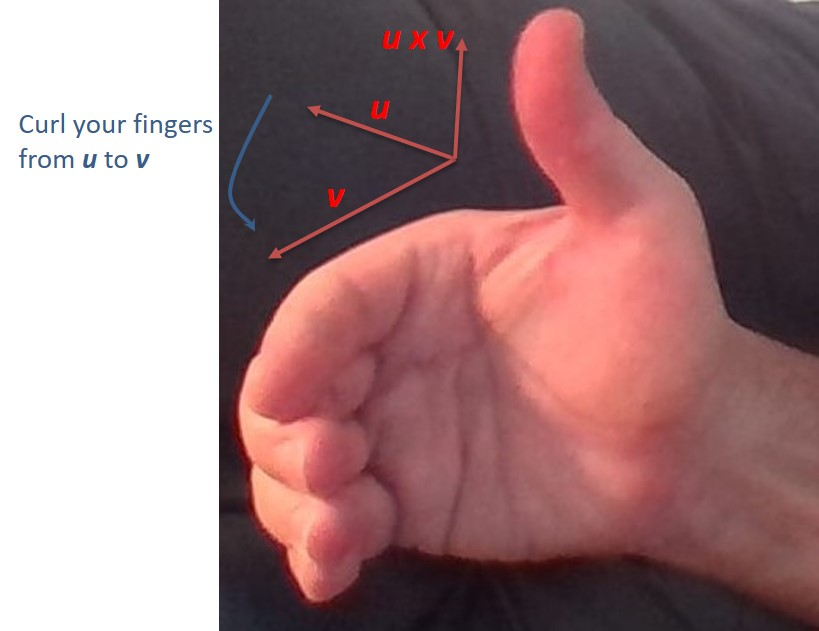Here are some vector products where you can use the right-hand rule to determine the direction of the vector product:$\bullet \quad \vec{\tau} = \vec{r} \times \vec{F}$ (torque)$\bullet \quad \vec{L} = \vec{r} \times \vec{p}$ (angular momentum)$\bullet \quad \vec{F}_B = q\vec{v} \times \vec{B}$ (magnetic force on a moving charge)$\bullet \quad \vec{F}_{wire} = I\vec{\ell} \times \vec{B}$ (magnetic force on a wire due to a current)

Use this same rule when you are constructing coordinate axes in space. Use the rule to point the three positive axes in the correct direction given that$\vec{x} \times \vec{y} = \vec{z}.$

• Magnetic field due to the current in a wire: When a current travels through a wire, it generates magnetic field lines that form concentric circles around the wire. But does the magnetic field point clockwise or counterclockwise? Use the right-hand rule to determine the direction of the field. Grip the wire with your right hand so that your thumb points in the direction of the (conventional) current. Then your fingers curl around the wire in the direction of the field. If the wire is bent into a loop, this same method tells you which direction through the loop the field points.In this photo, the current is moving to the left. Point your thumb to the left, and you see the field lines are moving down behind the wire and are moving up in front of the wire.

• Lenz’ law: When a loop of wire is placed in a location where the magnetic flux is changing, a current is induced in the wire. But in which direction is the induced current? Use a right-hand rule to determine the direction of the current. First determine the direction of the induced magnetic field predicted by Lenz’ law. If the flux is increasing through the loop the induced magnetic field has to point in the direction opposite the flux. If the flux is decreasing, the induced field points in the same direction as the flux. Now wrap your fingers around the wire so that they are pointing in the direction of this induced flux. Your thumb points in the direction of the induced current. Note that this right-hand rule is essentially the reverse of the previous rule.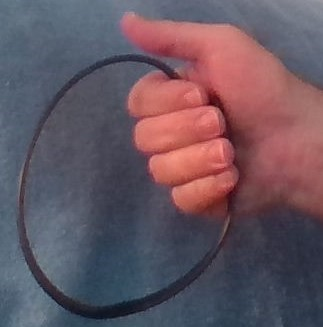In this photo, we have determined the induced flux must point out of the page towards our point of view. We curl our fingers to show this direction and we see the induced emf and induced current will be counterclockwise.

• Ampere’s law: The loop integral$\oint \vec{B} \cdot d \vec{s}$ around an Amperian loop is proportional to the net current through the loop. But in which direction through the loop is the current positive? Use a right-hand rule to determine the direction of positive current. Curl your fingers around the loop in the direction of integration. Your thumb points in the direction of positive current.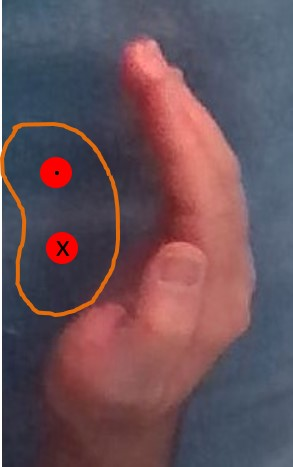Our Amperian loop is shown in orange in this photo. The two red circles represent current-carrying wires. The top wire has current coming out of the page and the bottom wire has current flowing into the page.  We choose to integrate in a counterclockwise direction. Curl your fingers in that direction and your thumb points out of the page (towards your point of view). Therefore the top wire will be assigned a positive current and the bottom wire will be assigned a negative current in order to apply Ampere’s law.

Physics

## Solving differential equations by separation of variables

A common differential equation is of the form$y' = f(x) \cdot g(y)$. In this situation, the equation can be solved by a technique called “separation of variables”. It involves putting all the y terms on one side of the equation and all the x terms on the other side. Then integration on both sides leads to a solution. Let’s look at a couple of examples.

Example 1

Solve:$\dfrac{dy}{dx} = \dfrac{2x}{y^2}$ with the initial condition$y(0) = 2.$

First, multiply both sides by y2 and by dx to separate the variables (all the y terms on one side and all the x terms on the other):$y^2 \; dy = 2x \; dx$

Note that dy/dx isn’t really a fraction, but you can treat it as a fraction to separate the variables. Now you have an exact differential on each side, so you can integrate both sides:$\int y^2 \; dy = \int 2x \; dx$$\dfrac{1}{3} y^3 = x^2 + C$

Why isn’t there a constant of integration on the left side? Well, there was, but we subtracted it and combined it with the constant of integration on the right so there is only one constant. Always do it this way, and put the constant on the independent variable side. Now solve for y:$y = \sqrt{3x^2 + C}$

Why didn’t we multiply the constant by 3? Well, we did, but a constant times three is still a constant, so we simplified it. Always keep your constant as simple as possible. Now solve for the constant, using the initial condition given:$2 = \sqrt{3(0)^2 + C} \rightarrow C = 8$$\therefore y = \sqrt{3x^2 + 8}$

Example 2:

Solve:$\dfrac{dy}{dx} = \dfrac{y}{\sqrt{x}}$ with the initial condition$y(0) = 2.$

As before, separate the variables:$\dfrac{dy}{y} = \dfrac{dx}{\sqrt{x}}$

Note that the dy and dx terms must always be in the numerator. Now integrate and solve for y:$\displaystyle \int \dfrac{dy}{y} = \int \dfrac{dx}{\sqrt{x}}$$\ln |y| = 2 \sqrt{x} + C$$y = e^{2 \sqrt{x} + C} = Ce^{2 \sqrt{x}}$

Wait, how did the constant move from the exponent to the coefficient? It’s a simplification trick. Proceed as follows:$y = e^{2 \sqrt{x} + C} = e^{2 \sqrt{x}} \cdot e^C$

But eC is a constant too, so just call it C. Where did the absolute value sign go? Because C can be either positive or negative, we can drop the absolute value sign around the y. Let’s finish the problem by solving for C:$2 = Ce^{2 \sqrt{0}} \rightarrow C = 2$$\therefore y = 2e^{2 \sqrt{x}}$

Calculus

## Tabular integration

Occasionally, you will be faced with a complicated integral such as$\int x^4 \cos 2x \; dx$

If you’ve read some of my other posts, you know that this requires integration by parts. Let’s get started on it and see where it leads.$\begin{matrix} u = x^4 & dv = \cos 2x \; dx \\ du = 4x^3 \; dx & v = \dfrac{\sin 2x}{2} \end{matrix}$

Then (after a bit of simplifying),$\int x^4 \cos 2x \; dx = \dfrac{1}{2} x^4 \sin 2x - 2 \int x^3 \sin 2x \; dx$

Now what? Well, the new integral requires integration by parts too! So you go through all the steps again. Then you have to do integration by parts on this result. And again on the next result! It’s tedious, but eventually you get to the correct answer. However, if one of the two functions in the original integral is a polynomial, there is a faster way to do this process. It’s called tabular integration, because all the parts of the integral are found by filling in a table.

Make a two-column table, with the columns labeled u and dv. Put the polynomial function (in our example, x4) under the u. Then take the derivative of this function and write it below the original function. Continue taking the derivative until you reach 0. In our example, your first column now looks like this.$\begin{matrix} \underline{u} & \underline{dv} \\ x^4 & \text{ } \\ 4x^3 & \text{ } \\ 12x^2 & \text{ } \\ 24x & \text { } \\ 24 & \text{ } \\ 0 & \text{ } \end{matrix}$

Next, place the other function in the dv column. Integrate this function repeatedly until there are entries in every row. In our example, your completed table will look like this:$\begin{matrix} \underline{u} & \underline{dv} \\ x^4 & \cos 2x \\ \text{ } \\4x^3 & \frac{1}{2} \sin 2x \\ \text{ } \\12x^2 & \frac{-1}{4} \cos 2x \\ \text{ } \\24x & \frac{-1}{8} \sin 2x \\ \text{ } \\24 & \frac{1}{16} \cos 2x \\ \text{ } \\0 & \frac{1}{32} \sin 2x \end{matrix}$

You’ve done all the hard work! Now you can write out the integral from the values in this table. First draw an arrow from each u value (except 0) to the dv value on the next line below, as shown here: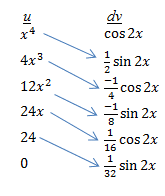Next, place alternating plus and minus signs on each arrow: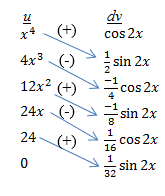Now multiply each u value by the dv value at the other end of the arrow. The plus or minus sign tells you whether to add or subtract this term. For this problem, the integral would be:$(x^4)(\frac{1}{2} \sin 2x) - (4x^3)(\frac{-1}{4} \cos 2x) + (12x^2)(\frac{-1}{8} \sin 2x) - (24x)(\frac{1}{16} \cos 2x) + (24)(\frac{1}{32} \sin 2x)$

Simplifying gives the final answer (don’t forget the C!):$\frac{1}{2} x^4 \sin 2x \; + \; x^3 \cos 2x \; - \; \frac{3}{2} x^2 \sin 2x \; - \; \frac{3}{2} \cos 2x \; + \; \frac{3}{4} \sin 2x \; + \; C$

Calculus

## How does the speed of light relate to Maxwell’s equations?

You may have seen in your physics class that the speed of light can be calculated from the permittivity of free space,$\varepsilon_0$, and the permeability of free space,$\mu_0$, as follows:$c = \dfrac{1}{\sqrt{\varepsilon_0 \mu_0}}$

where$\varepsilon_0 = 8.85 \; C^2/N \cdot m^2$ and$\mu_0 = 4 \pi \cdot 10^{-7} \; T \cdot m/A$ .

Sure enough, plug the numbers into your calculator and you do get the correct value for the speed of light. It’s a very famous result, and helped convince Maxwell that light is an electromagnetic wave. But the units just don’t seem to work out, do they? Well, actually they do. Let’s do the dimensional analysis. We want to show the following:$\dfrac{m}{s} = \dfrac{1}{\sqrt{\dfrac{C^2}{N \cdot m^2} \times \dfrac{T \cdot m}{A}}} \; \; (1)$

From the equation for the magnetic force on a charged particle:$F = qvB \; \rightarrow \; B = \dfrac{F}{qv}$

If we express this equation in terms of its units and simplify we have$T = \dfrac{N}{C \cdot m/s} = \dfrac{N \cdot s}{C \cdot m}$

We also note that 1 A = 1 C/s, so we substitute for T and A in equation (1) above to get:$\dfrac{m}{s} = \dfrac{1}{\sqrt{\dfrac{C^2}{N \cdot m^2} \times \dfrac{N \cdot s}{C \cdot m} \dfrac{m \cdot s}{C}}} = \dfrac{1}{\sqrt{\dfrac{s^2}{m^2}}} = \dfrac{m}{s}$

Physics

## finding the number of diagonals in a polygon

How many diagonals are there in an octagon?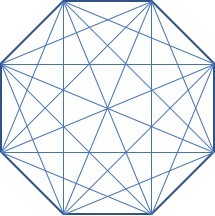Instead of trying to count them all, use the formula for the number of diagonals in any polygon:$d= \dfrac{n(n-3)}{2}$

where n = the number of sides and d= the number of diagonals. For an octagon, n= 8, so$d= \dfrac{8(8-3)}{2} = 20$

This is a good formula to memorize and its derivation is pretty simple, so let’s see it. We will use the same octagon diagram, but only draw the diagonals from one vertex: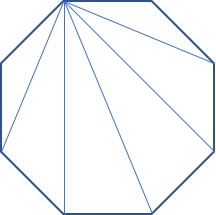You can see that from this vertex on the upper left, a diagonal can be drawn to every vertex except the two adjacent vertices and to itself. So the number of diagonals from any vertex in an n-gon is (n-3). Since there are n vertices, the total number of diagonals is n(n-3). But we’ve just counted every diagonal twice (once from each endpoint), so to find the number of different diagonals, we divide by two. This gives us the formula above.

Geometry

## finding internal or external angles of regular polygons

How do you find the measure of an internal angle or an external angle on a regular polygon? It’s quite easy, if you memorize two formulas. All you have to do is count the number of sides on the polygon and plug it into the formula.

For internal angles, use the formula$\alpha = \dfrac{180(n-2)}{n}$

For external angles, the formula is even easier:$\beta = \dfrac{360}{n}$

For example, here is a regular octagon, so n = 8. The internal angle is labeled α and the external angle is β.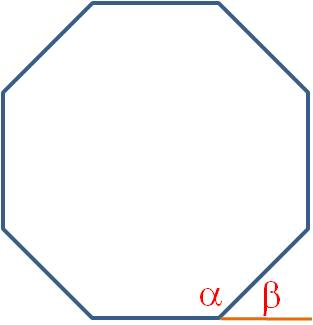$\alpha = \dfrac{180(8-2)}{8}=135 \textdegree$$\beta = \dfrac{360}{8} = 45 \textdegree$

And here’s a trick to save you some time: You can see in the figure that the internal and external angles are always supplementary. (This is true in any polygon, even if it isn’t regular.) So if you need to find the internal angle, you can find the external angle instead with the simpler formula. Then the supplement to that angle is the internal angle!

Geometry

## Calculating permutations and combinations

When counting up the number of ways an event can occur, you use the formulas for permutations and combinations. You should be familiar with the nPr and nCr commands on your calculator, and this is the easiest way to evaluate these problems. But if your calculator doesn’t have these functions, there is a fairly simple way to set up these operations. This is the way we had to calculate permutations and combinations when calculators did not have these functions built in. Practice a couple of these examples and you’ll see that you can calculate permutations and combinations almost as quickly as your calculator can do it.

Calculating nPr

To calculate nPr, you will multiply together r consecutive numbers, starting with n and counting down. For example, 12P 3 is equal to 12*11*10 = 1320. We started with 12 (the value of n) and counted down to 10 so that we had 3 numbers (3 is the value of r). As another example, 7P5 = 7*6*5*4*3 = 2520.

Calculating nCr

To calculate nCr, create a fraction. The numerator is the same as above; that is, start with n and count down r consecutive numbers. The denominator is the smaller of r! and (n-r)!. For example,12P3 is$\dfrac{12*11*10}{1*2*3}$

Before you calculate this fraction, simplify it. All of the terms in the denominator will always cancel out with terms in the numerator, leaving you with just numbers in the numerator to multiply together. For example,$\dfrac{12*11*10}{1*2*3} = 2 * 11 * 10 = 220$

To calculate 7C5, note that 7C5 = 7C2 . Then,$\dfrac{7*6}{1*2} = 7 * 3= 21$

## Calorimetry calculations II—measuring heats of reactions

In my previous post, I showed you how to work a calorimetry problem when you drop a hot block of metal into the calorimeter. In this post, we will look at the heat of reaction problem. The principles are the same: all of the heat released or absorbed by the reactants is absorbed or released by the water. In formula terms, this is written as ΔH = -q. From the M-CAT equation we examined in the previous post, we can write this as$\Delta H_{rxn} = -m_{solution} c_{solution} \Delta T_{solution}$

We use this equation to find the molar heat of reaction as shown here:

Example: The temperature of a beaker containing 150 mL of water drops by 3.85°C when 8.00 g of ammonium nitrate are added. Determine the molar heat of solution for ammonium nitrate. Assume the specific heat capacity of the solution is 4.18 J/g·°C.

Answer: The heat of solution is defined as the ΔH for the following reaction:$NH_4NO_3 \; (s) \rightarrow NH_4^+ \; (aq) + NO_3^- \; (aq)$

The mass of 150 mL of water is 150 g. Therefore the total mass of the solution is 158 g. So$\Delta H_{rxn} = -(158)(4.18)(-3.85) = 2540 \; J$

The molar mass of ammonium nitrate is 80.0 g, so the molar heat of solution is$\dfrac{2540 \; J}{8.00 \; g \; NH_4NO_3} \times \dfrac{80.0 \; g}{1 \; mol} = 25.4 \; kJ/mol$

Notes: We must include the mass of the ammonium nitrate in the M-CAT equation because it cools down along with the water. We also assumed the specific heat capacity of the solution was the same as the specific heat capacity of pure water. For dilute solutions, this is a reasonable assumption.

Chemistry

## Calorimetry calculations I—dropping hot blocks into water

Calorimetry refers to the measure of how much heat is transferred in a reaction or phase change. We measure this heat with a device called a calorimeter. Although serious chemists will use serious calorimeters costing hundreds or thousands of dollars, you can duplicate much of their work by pouring some water into a Styrofoam cup. Your answers won’t be as precise as the serious chemists’ answers, but for two or three sig figs, this method is good enough.

There are two kinds of calorimetry problems; we’ll look at one here and the other in the next post. The first involves dropping a heated block of metal into the cup of water. The block cools down and the water warms up until the block and water are at the same temperature (thermal equilibrium). In the second type of problem, a chemical reaction takes place in the water. If the reaction is exothermic, the water absorbs the heat and warms up. If the reaction is endothermic, heat is absorbed from the water and the water cools down.

Let’s examine the first problem: There are two guiding principles we observe. The first is that heat will flow from the hot block into the water until the block and the water are at the same temperature. The second principle is that (we assume) there is no loss of heat to the air. In other words, the heat lost by the block exactly equals the heat absorbed by the water.

Both the heat lost by the block and the heat the water absorbs are governed by a simple equation:$q = mc \Delta T$

Here q is the amount of heat gained or lost, m is the mass of the substance, c is the specific heat capacity of the substance, and ΔT is the change in temperature. Many teachers call this the M-CAT equation because the Δ looks like an A if you squint really hard. If this helps you remember it, you can call it that too. The value of c, the specific heat capacity, is a constant for a given substance. It measures how much heat must be absorbed by 1 g of a material to raise its temperature by 1°C. You might be asked to memorize the specific heat capacity of water. It is 1.00 cal/g·°C or 4.18 J/g·°C. You probably will not need to memorize specific heat capacity values for any other substance.

With the equation, calorimetry problems of this type are simple algebra problems. We have$q_{water} = m_{water} c_{water} \Delta T_{water}$ and$q_{block} = m_{block} c_{block} \Delta T_{block}$. Also, since the heat lost by the block equals the heat gained by the water, we can write$q_{water} = -q_{block}$. Therefore,$m_{water} c_{water} \Delta T_{water} = -m_{block} c_{block} \Delta T_{block}$ .

This is our magic equation. There are two kinds of questions you will answer with this equation. In one, we tell you the initial and equilibrium temperatures and you calculate the specific heat capacity of the block substance. In the other, you are given the initial temperatures and the specific heat of the block and you calculate the equilibrium temperature. Here are examples that show both types:

Example 1: A 25 g block of aluminum is heated to 85°C and placed into a calorimeter with 80 g of water at 25°C. The final temperature of the calorimeter and block is 28.8°C. Calculate the specific heat of aluminum.

Solution 1: We calculate the ΔT of the water (3.8°C) and the aluminum block (-56.2°C) and plug everything into the equation above.

(80)(4.18)(3.8) = -(25)(c)(-56.2). Solving gives c = 0.90 J/g·°C.

Example 2: A 25 g block of aluminum is heated to 85°C and placed into a calorimeter with 80 g of water at 25°C. The specific heat of aluminum is 0.90 J/g·°C. What is the equilibrium temperature of the calorimeter?

Solution 2: This problem (really just a rewording of example 1) is only a little more complicated to solve than example 1. First note that ΔT = Tf – Ti. We make this substitution and our magic equation becomes

(80)(4.18)(Tf – 25) = -(25)(0.90)(Tf – 85)

Distribute and simplify to get 334.4 Tf – 8360 = 1912.5 – 22.5 Tf. Solving gives Tf = 28.8°C.

Note: This is one of the rare times you do not need to convert the temperature from °C to kelvins. This is because the temperature term in the equation is a change in temperature and not an absolute temperature. Changing the temperature by 1°C is the same as changing it by 1 K.

Chemistry
Blue Taste Theme created by Jabox